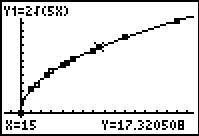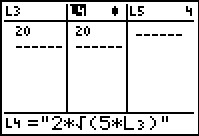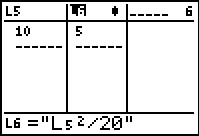••• ##### Device
• TI-83 Plus Family
• TI-84 Plus
• TI-84 Plus Silver Edition
•TI-84 Plus C Silver Edition
•TI-84 Plus CE
• ##### Software

TI Connect™
TI Connect™ CE

# Algebra 1: STOP

by Texas Instruments - Bell Ringer Lesson#### Overview

Students use an interactive page to calculate the speed of the car, given a stopping distance, and then approximate stopping distance, given the rate of the car.

#### Key Steps

•After an introduction/review of converting between miles per hour and meters per second, students will use a formula in a list to calculate the speed of the car, given a stopping distance.

•Then students will use the same formula to find an approximate stopping distance, given the rate of the car.

•Students use both the lists and scatter plot to make some observations about how the stopping distance and speed of the car are related. Finally, the students will graph the formula as a function to see the general trend of the data and are introduced to a Square Root function.